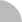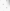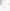(35.172.233.215) 您好！臺灣時間：2020/08/13 00:25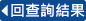### 詳目顯示:::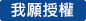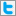Twitter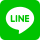•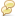被引用:0
•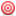點閱:204
•評分: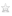•下載:0
•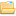書目收藏:1
 約在1913年左右 Ramanujan 猜想方程式x^2+7=2^n只有五組正整數解，此猜想在1948年由Nagell首先給出證明，其後有釵h數學家以不同的方法再一次證明此猜想。在1980年以後，釵h數學家開始研究方程式D_1x^2+D_2=λ^2k^n 的正整數解。在余茂華一系列的論文中證明除了方程式x^2+7=2^(n+2)有五組正整數解及方程式3x^2+5=2^(n+2)、x^2+11=2^2×3^n、x^2+19=2^2×5^n各有三組正整數解外，方程式D_1x^2+D_2=λ^2k^n 的正整數解個數最多二組。此篇論文主要討論方程式2x^2+1=3^n的正整數解，由余茂華之前的結果得到方程式2x^2+1=3^n的正整數解個數最多二組，而在Begeard 和 Sheory 的論文中給定此方程式的正整數解分別為(x,n)=(1,1)及(2,2)，但是除了n=1,2，我們發現n=5也是此方程式的一個解。在此篇論文中，我們將求正整數解的問題轉換成二次遞迴數列(binary recurrent sequence)的問題並且利用Beukers論文中的相關結果來證明方程式2x^2+1=3^n僅有三組正整數解(x,n)=(1,1)，(2,2)以及(11,5)。
 Ramanujan observed in 1913 that1^2 + 7 = 2^3 , 3^2 + 7 = 2^4 , 5^2 + 7 = 2^5 ,11^2 + 7 = 2^7 , (181)^2 + 7 = 2^15 .(1.1)When looking for the solutions of the equationx^2 + 7 = 2^n in integers x > 0, n > 0,(1.2)Ramanujan conjectured in 1913 that all the solutions of equation(1.2) are given by (1.1). This was proved by Nagell in 1948 and by others, using several different proofs. The equation (1.2) is called theRamanujan-Nagell equation and has applications to binary error-correcting codes.After 1980’s, many mathematicians concentrated on studying the equationD_1x^2 + D_2 =λ^2 k^n, (1.3)We denote by N(λ, D_1, D_2, k) the number of solutions(x, n) of the equation (1.3). For D_1 = 1, equation (1.3) is usuallycalled the generalized Ramanujan-Nagell equation. In a series of papers, Le proved that N(λ,D_1, D_2,p)≦2 except forN(2, 1, 7, 2) = 5 and N(2, 3, 5, 2) = N(2, 1, 11, 3) = N(2, 1, 19, 5) =3. Bugeaud and Shorey offer the newest results and related ref-erencesabout equation (1.3).In this paper, we are looking for solutions of the equation 2x^2 + 1 = 3^n in integer x≧1, n≧1. (1.4)The previous result of Le implies N(1, 2, 1, 3) ≦2, and Bugeaud and Shorey refer that equation (1.4) has two solutions which aregiven by n = 1, 2. But except for n = 1, 2, we find n = 5 is also a solution of equation (1.4). In this paper we will reduce the problemof determining N(1, 2, 1, 3) to a binary recurrent sequence and use the results of Beukers  to prove that the equation (1.4) has onlythe solutions (x, n) = (1, 1), (2, 2) and (11, 5).
 1 Introduction2 Preliminaries3 The Equation 2x^2+1=3^nReferences
 1. F. Beukers, The Multiplicity of Binary Recurrences, CompositioMath. 40 (1980), no. 2, 251-267.2. Y. Bugeaud and T. N. Shorey, On the number of solutions of thegeneralized Ramanujan-Nagell equation, J. reine angew. Math.539 (2001), 55-74.3. E. L. Cohen, The Diophantine Equation x^2 +11 = 3^k and RelatedQuestions, Math. Scand. 38 (1976), no. 2, 240-246.4. T. W. Hungerford, Algebra, Springer-Verlag, 1974.5. W. Johnson, The Diophantine Equation x^2 + 7 = 2^n , Amer.Math. Monthly 94 (1987), no. 1, 59-62.6. Maohua Le, The divisibility of the class number for a class ofimaginary quadratic fields, Kexue Tongbao 32 (1987), no. 10,724—727.(in Chinese)7. Maohua Le, On the number of solutions of the diophantine equa-tionx^2 + D = p^n , C. R. Acad. Sci. paris S´er. A 317 (1993),135-138.8. Maohua Le, On the Diophantine Equation D_1x^2 + D_2 = 2^(n+2),Acta Arith. 64 (1993), 29-41.9. Maohua Le, A note on the Generalized Ramanujan-Nagell Equation,J. Number Th. 50 (1995), 193-201.10. Maohua Le, A Note on the Number of Solutions of the GeneralizedRamanujan-Nagell Equation D_1x^2 + D_2 = 4p^n , J. NumberTh. 62 (1997), 100-106.11. Maohua Le, On the Diophantine Equation (x^3− 1)/(x − 1) =(y^n− 1)/(y − 1), Trans. Amer. Math. Soc. 351 (1999), 1063-1074.12. D. A. Marcus, Number fields, Springer-Verlag, 1987.13. T. Nagell, The Diophantine Equation x^2 + 7 = 2^n , Ark. Mat. 4(1961), 185-187.14. I. Niven, H. S. Zuckerman, An Introduction to the Theory ofNumbers, 4th ed., John Wiley and Sons, 1980.15. S. Ramanujan, Collected Papers, Chelsea Publishing Co., NewYork, 1962, 327.16. H. S. Shapiro and D. L. Slotnick, On the mathematical theoryof error-correcting codes, IBM J. Res. Develop. 3 (1959), 25-34.17. Th. Skolem, S. Chowla and D. J. Lewis, The Diophantine equation2^(n+2)− 7 = x^2 and related problems, Proc. Amer. Math.Soc. 10 (1959), 663—669.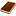國圖紙本論文推文當script無法執行時可按︰推文 網路書籤當script無法執行時可按︰網路書籤 推薦當script無法執行時可按︰推薦 評分當script無法執行時可按︰評分 引用網址當script無法執行時可按︰引用網址 轉寄當script無法執行時可按︰轉寄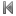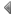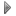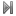top
 無相關論文

 無相關期刊

 1 二次西格模形式的一些性質 2 奇異積分算子的加權模不等式 3 非線性邊界值問題正解之探討 4 迴圈之冪圖的星林分解數 5 統計模型參數和之估計 6 加權赫茲形式哈弟空間上的郝曼德乘算子 7 二組件組合產品之故障率的非母數估計 8 非線性常微分方程式之邊界值問題的討論 9 物件導向設計在複合區域網格生成系統的應用 10 雙競爭物種的非平面波 11 具非局部項之反應擴散方程的週期行波解 12 具時間延遲合作型Lotka-Volterra系統之行進波解 13 Kolmogorov-Fisher型態的反應擴散方程 14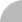簡易查詢 | 進階查詢 | 論文瀏覽 | 熱門排行 | 我的研究室